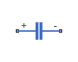# Capacitor

Linear capacitor in electrical systems

• Library:
• Simscape / Foundation Library / Electrical / Electrical Elements

•## Description

The Capacitor block models a linear capacitor, described with the following equation:

`$I=C\frac{dV}{dt}$`

where:

• I is current.

• C is capacitance.

• V is voltage.

• t is time.

The Series resistance and Parallel conductance parameters represent small parasitic effects. The parallel conductance directly across the capacitor can be used to model dielectric losses, or equivalently leakage current per volt. The series resistance can be used to represent component effective series resistance (ESR) or connection resistance. Simulation of some circuits may require the presence of the small series resistance. For more information, see Modeling Best Practices.

Connections + and – are conserving electrical ports corresponding to the positive and negative terminals of the capacitor, respectively. The current is positive if it flows from positive to negative, and the voltage across the capacitor is equal to the difference between the voltage at the positive and the negative terminal, V(+) – V(–).

### Variables

To set the priority and initial target values for the block variables prior to simulation, use the Initial Targets section in the block dialog box or Property Inspector. For more information, see Set Priority and Initial Target for Block Variables.

Nominal values provide a way to specify the expected magnitude of a variable in a model. Using system scaling based on nominal values increases the simulation robustness. Nominal values can come from different sources, one of which is the Nominal Values section in the block dialog box or Property Inspector. For more information, see Modify Nominal Values for a Block Variable.

## Ports

### Conserving

expand all

Electrical conserving port associated with the capacitor positive terminal.

Electrical conserving port associated with the capacitor negative terminal.

## Parameters

expand all

Capacitance value.

Represents small parasitic effects. The series resistance can be used to represent component internal resistance, effective series resistance (ESR), or connection resistance. Simulation of some circuits may require the presence of the small series resistance.

Represents small parasitic effects. The parallel conductance directly across the capacitor can be used to model dielectric losses, or leakage current per volt.

## Version History

Introduced in R2007a